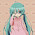## 2015年1月16日 星期五

### [smart pointer] [reference counter] 智能指標 引用計數

reference_pointer<int> a;//建立一個int的引用指標a
a = new_reference(5);//a指向新增int動態變數，其值為5
a = new_reference<int>(5);//同上，只是定義較為嚴謹
a = new_reference((int)5);//同上，只是定義較為嚴謹
reference_pointer<int> b = a;//將b指向a所指向之物

struct P{
int a,b;
P(int _a,int _b):a(_a),b(_b){}
}p(2,3);
reference_pointer<P> a;//建立一個P的引用指標a
c = new_reference(P(1,2));//指向新增P動態變數，其值為1,2
c = new_reference<P>(P(1,2));//同上，只是定義較為嚴謹
c = new_reference(p);//指向新增P動態變數，其值為p


#### 1 則留言:

1.